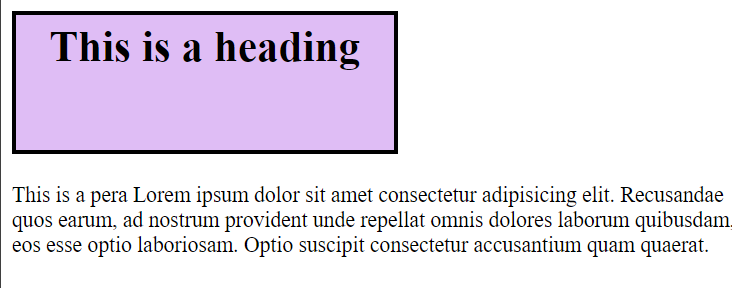CSS Math Functions

# CSS Math Functions

CSS Math Functions
We use mathematical functions in css when we want to use mathematical expressions as CSS properties.
Accepts a mathematical expression and returns a numerical value.
It is especially useful when working with different types of units (e.g. subtracting a px value from a percentage) to calculate the value of an attribute.
+, -, /, and * operators can all be used, and parentheses can be added to specify the order of operations if necessary.
Basically there are three commonly used mathematical functions given as follows -
1. calc()
2. max()
3. min()
Now we will see all the three one by one -
1. Calc()
The calc function lets you combine the different units we saw earlier to calculate an exact amount.
It can be used anywhere a value is expected.
The real power of the calc function is that you can have mixed units in the calculation.
Syntax -
 calc(expression)
Now we will see example -
Example -
Use calc() to determine the height of an element -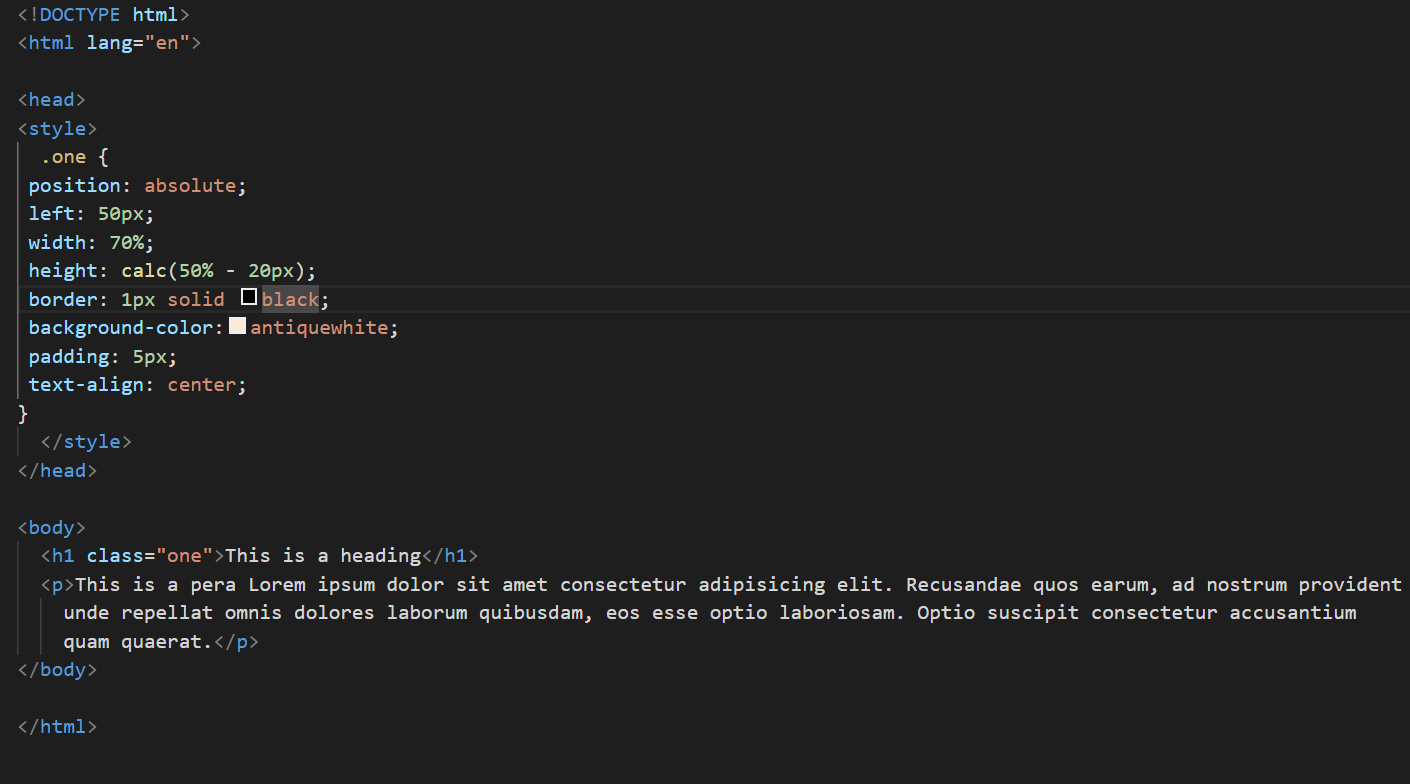Output -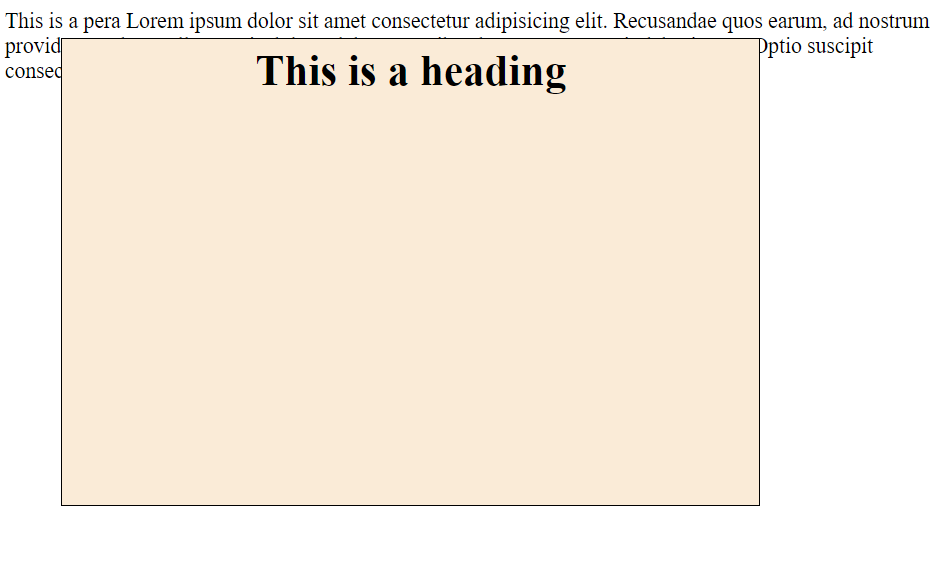2. max()
This function uses the max value from the given values separated by a comma in the property value.
Syntax -
 max(value1, value2, ...)
Now we will see Example -
Example -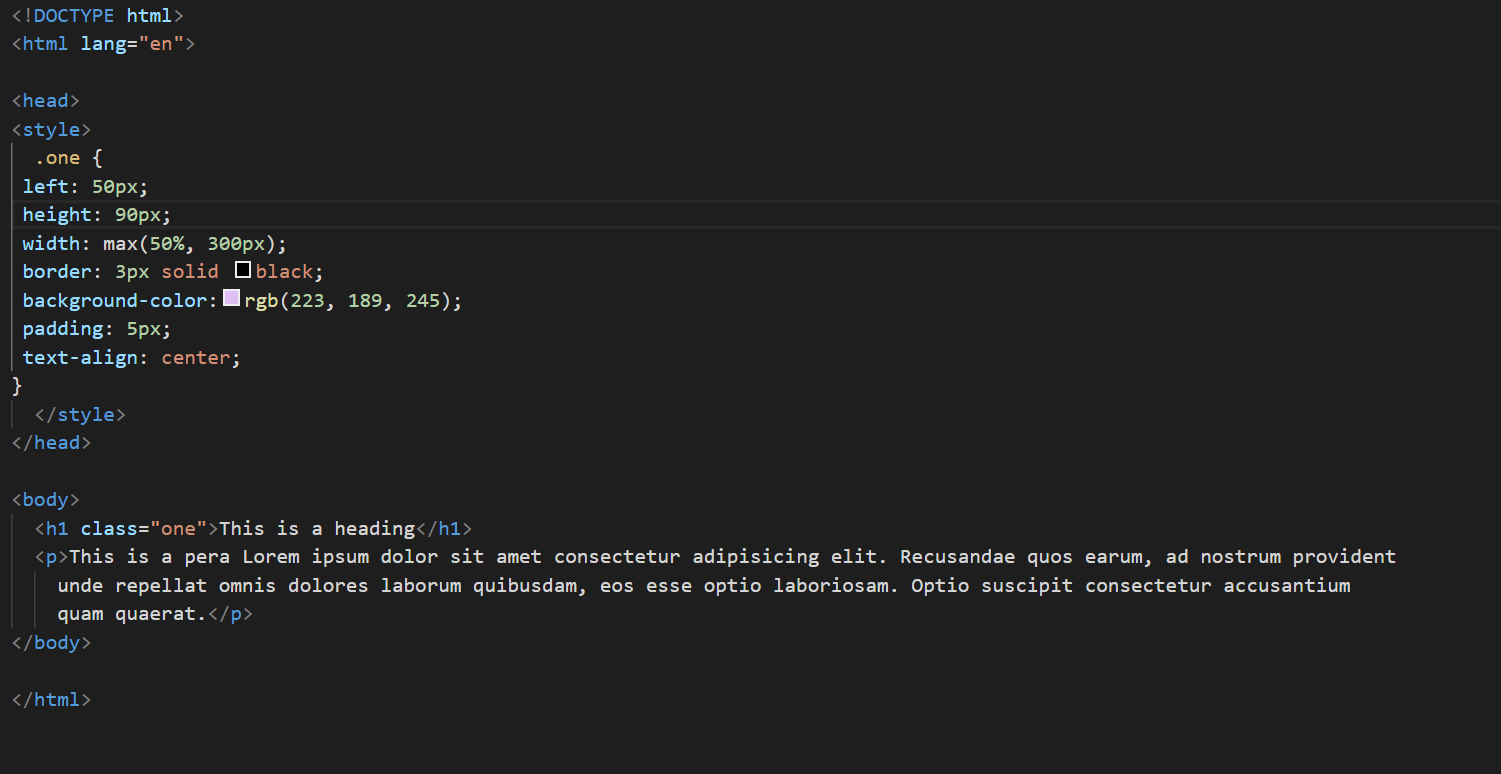Output -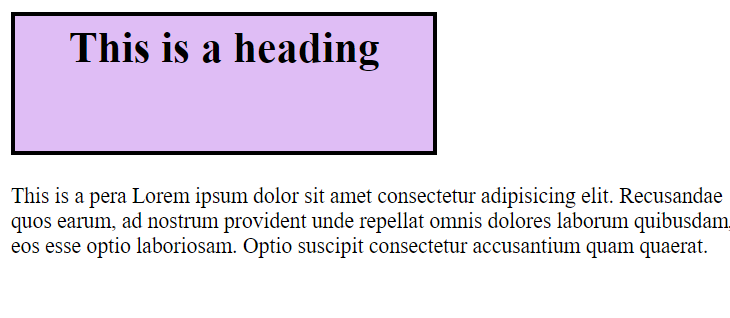3. min()
This function uses the min value from the given values separated by a comma in the property value.
Syntax -
 min(value1, value2, ...)
Now we will see Example -
Example -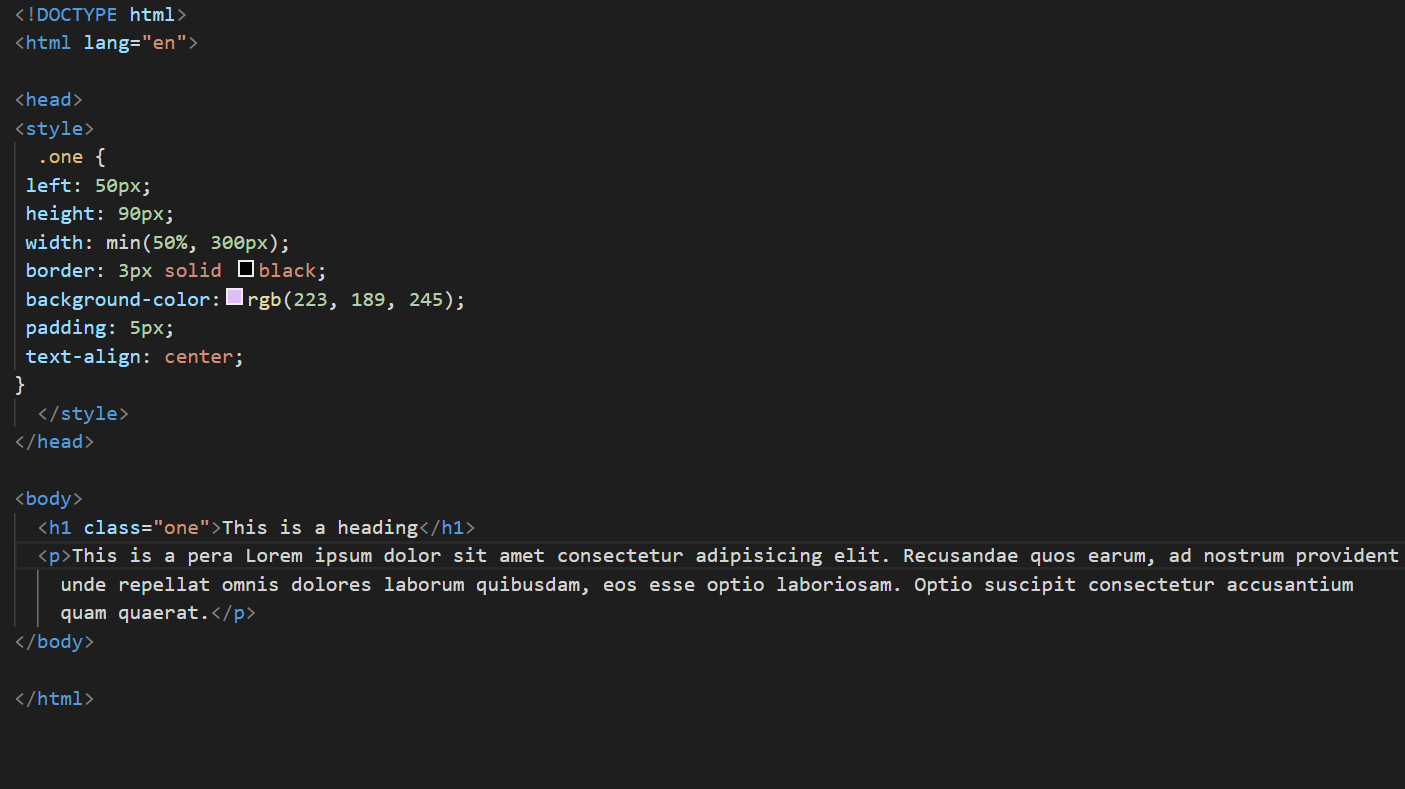Output -